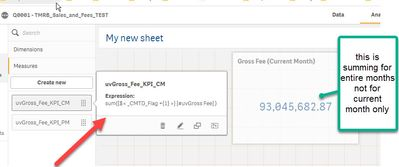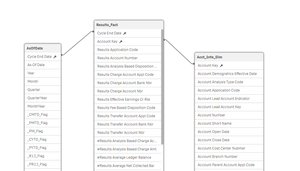# App Development

Announcements
cancel
Showing results for
Did you mean:Contributor III

## KPI not giving me sum for the current month.

Hello,

I am just a beginner and I am looking for help in set operators using conditional flag.

I have a simple star schema (I have provided the model image below). I have generated the master colander for deterring current month and previous month flags.

I have a measure called #uvGrossFee, which I need to sum up for the current month to display as KPI for the current month.

I am using set operators as follows:

sum({\$< _CMTD_Flag ={1} >} [#uvGross Fee] )

-- This is summing up and giving me total for the entire months. I am looking for the summation of gross fees for the current month only.  Please note I am using this as KPI and I don't want its value to be changed with any dimension selection from any filter pane (for now, i have not used any filter pane).

I even tried this:

sum({\$< asofdate=, _CMTD_Flag ={1} >} [#uvGross Fee] )

Can someone please advise what I am missing here?Below is the model I have developed./*** Below is the script I have used for developing Mater Table **/ Source "QlikView Your Business"

Temp_Dates:
FieldValue('Cycle End Date', RecNo()) as "Cycle End Date"
AutoGenerate
FieldValueCount('Cycle End Date')
;

Join (Temp_Dates)

Date(floor(MonthEnd(AddMonths(MinDate, IterNo() -1)))) as [As Of Date]
WHILE
;

Date(floor(MonthEnd(Min("Cycle End Date")))) as MinDate,
Date(floor(MonthEnd(Max("Cycle End Date")))) as MaxDate
resident
Temp_Dates
;

AsOfDate:
"Cycle End Date",
[As Of Date] ,
Year([As Of Date] ) AS Year,
Month([As Of Date] ) AS Month,
'Q' & ceil(month([As Of Date] ) / 3) AS Quarter,
Year([As Of Date] ) &'-'& 'Q' & ceil(month([As Of Date] ) / 3)
AS QuarterYear,

Date([As Of Date] , 'MMM-YYYY') AS MonthYear,

InMonth("Cycle End Date", [As Of Date] , 0) * -1 AS _CMTD_Flag,
InMonth("Cycle End Date", [As Of Date] , -12) * -1 AS _PMTD_Flag,
InMonth("Cycle End Date", [As Of Date] , -1) * -1 AS _PM_Flag,

InYearToDate("Cycle End Date", [As Of Date] , 0) * -1 AS _CYTD_Flag,
InYearToDate("Cycle End Date", [As Of Date] , -1) * -1 AS _PYTD_Flag,
(AddMonths("Cycle End Date", 12) > [As Of Date] ) * -1 AS _R12_Flag,
(AddMonths("Cycle End Date", 12) <= [As Of Date] ) *
(AddMonths("Cycle End Date", 24) > [As Of Date] ) AS _PR12_Flag,

(AddMonths("Cycle End Date", 6) > [As Of Date] ) * -1 AS _R6_Flag,

(AddMonths("Cycle End Date", 6) <= [As Of Date] ) *
(AddMonths("Cycle End Date", 12) > [As Of Date] ) AS _PR6_Flag,

(AddMonths("Cycle End Date", 12) <= [As Of Date] ) *
(AddMonths("Cycle End Date", 18) > [As Of Date] ) AS _PYR6_Flag,

(AddMonths("Cycle End Date", 3) > [As Of Date] ) * -1 AS _R3_Flag,

(AddMonths("Cycle End Date", 3) <= [As Of Date] ) *
(AddMonths("Cycle End Date", 6) > [As Of Date] ) AS _PR3_Flag,

(AddMonths("Cycle End Date", 12) <= [As Of Date] ) *
(AddMonths("Cycle End Date", 15) > [As Of Date] ) AS _PYR3_Flag

resident
Temp_Dates
where
"Cycle End Date"<=[As Of Date]
;

drop table Temp_Dates;

4 RepliesMVP

InMonth("Cycle End Date", [As Of Date] , 0) * -1 AS _CMTD_Flag,

LET vMaxDate = Num(Today()-1); // assign the max date based on ur requirement

InMonthToDate("Cycle End Date",\$(vMaxDate),0) * -1 as _CMTD_Flag,

Thanks & Regards,
Mayil Vahanan RContributor III
Author

Hi Mayil,

Thanks for getting back to me. But, I cant use vMaxDate as Today() because I want to have flexibility in data load. For example, I might load the data only till December 2020 instead of February 2021. In such case current month should be December 2020 not February 2020.

Looks like no matter what, I need to use the As of Date from Filter Pane. On that basis only the KPI will work for that given month.

Please let me know if you think otherwise.

Thanks again.

BinayMVP

Try like below

sum({\$< [As Of Date] ={">=\$(=date(MonthStart(Max([As Of Date]),0),'DD/MM/YYYY'))<=\$(=Date(MonthEnd(Max([As Of Date]),0),'DD/MM/YYYY'))"} >} [#uvGross Fee] )

Thanks & Regards,
Mayil Vahanan RContributor III
Author

This approach should work. Let me try.